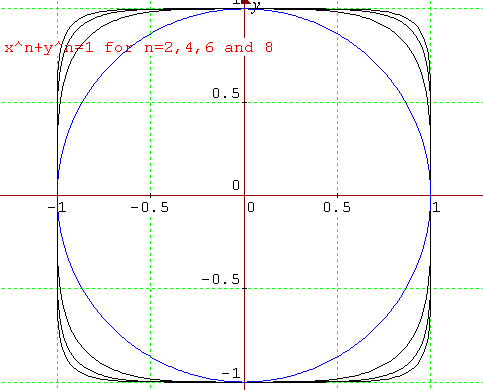#### You may also like### Degree Ceremony

What does Pythagoras' Theorem tell you about these angles: 90Â°, (45+x)Â° and (45-x)Â° in a triangle?### After Thought

Which is larger cos(sin x) or sin(cos x) ? Does this depend on x ?### Small Steps

Two problems about infinite processes where smaller and smaller steps are taken and you have to discover what happens in the limit.

# Squareness

##### Age 16 to 18Challenge LevelThis question is about the family of relations given by $x^n+y^n=1$

(1) Explain the features of the graph of the relation $|x|+|y|=1$.

(2) Prove that $${n\over n+1} \leq {1\over 2^{1/n}} < 1$$

(3) Consider the family of relations $x^n+y^n=1$ in the first quadrant.

Choose one particular value of $n$ and show that $y$ decreases as $x$ increases.

Show that, for each value of $n$, the graph lies entirely outside the square bounded by the lines $$x=0, \ x={n\over n+1},\ y=0,\ y={n\over n+1}$$ and inside the square bounded by the lines $$x=0,\ x=1,\ y=0,\ y=1.$$

(4) Sketch some graphs in all four quadrants of the family of relations $|x|^n+|y|^n=1$ for even values of $n$ and explain why the graphs get closer to a square shape as $n\to \infty$.

(5) Plot the graph of $x^3+y^3=1$ in all four quadrants. Why do the graphs of the relations $x^n+y^n=1$ differ according to whether $n$ is odd or even?